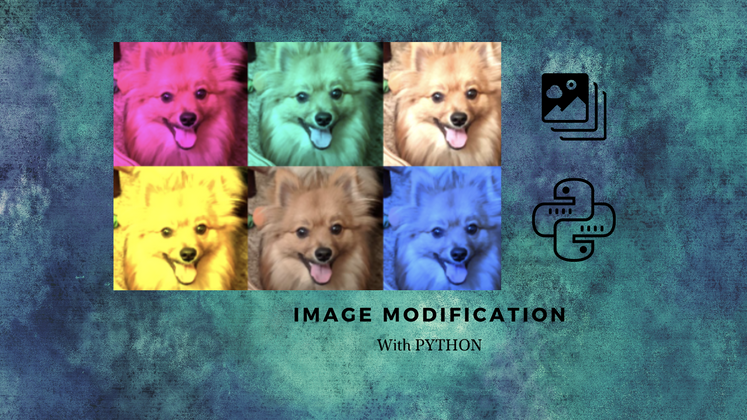## CodeNewbie Community is a community of 5,745 amazing newbiesPosted on • Originally published at bharadwaj.hashnode.dev on

# Image modification using SimpleImage library!

Hello all!! How are you doing? Hope you all are doing well. Let's get straight into the topic. In this blog, I am going to talk about image modification in Python using the SimpleImage library.

We all know that images are made of square pixels. Every pixel has (x,y) coordinates in the image. The origin(0,0) is at the upper-left corner. Y increases going down, X increases going up.

Each pixel has a single color encoded as RGB values. Each value represents brightness for that color. Each RGB value range from 0 to 255. We can set RGB values to make any color.

if RGB = (255,255,255) then it is white in color. If it is (0,0,0) then there is an absence of light in all three colors. So, it is black in color.

Installing Pillow

Pillow is the version of Python Imaging Library(PIL). In this library, we use SimpleImage to modify our images.

For Windows:

``````py -m pip install Pillow

``````

For mac:

``````python3 -m pip install Pillow

``````

Using Simple Image library

To use any library in python, we should import it. And we should include it at top of our program file.

``````from simpleimage import SimpleImage

``````

Functions in SimpleImage library

A. Create a SimpleImage object by reading an image from a file(jpg, png, gif, etc.) and store it in a variable.

``````my_image = SimpleImage(filename)

``````

B. Show image on your computer

``````my_image.show

``````

We can manipulate an image by changing its pixels. We can also create new pixels and set their pixels.

Accessing pixels in an Image:

We use a new kind of loop called the for-each loop.

``````for-each loop in image:
image = SimpleImage("flower.png")
for pixel in image:
#Do something

``````

Properties of images and pixels:

• Each SimpleImage has properties you can access. They are image.width, image.height.
• Each pixel in the image has properties. pixel.x, pixel.y, pixel.red, pixel.blue, pixel.green.
• Higher R, G, B values mean more of the color in pixel.
• Pixels are mutable.
• We can set pixel RGB values in an image in order to change it.

Let's solve some examples from the concepts we learned.

A. Making image darker

Here all the colors of the images stand as they are, but are reduced by some pixels. (Light fall on the images are reduced).

``````def darker(image):
for pixel in image:
pixel.red = pixel.red // 2
pixel.green = pixel.green // 2
pixel.blue = pixel.blue // 2
def main():
flower = SimpleImage('flower.png')
darker(flower)
flower.show()

``````

B. Extracting Red channel from the image

All colors other than red are reduced to zero. So, there is no light other than red in the image.

``````def red_channel(filename):
img = SimpleImage(filename)
for px in image:
px.red = pix.red // 2
px.green = 0
px.blue = 0
return img

``````

C. Greyscale

Calculates the luminosity of a pixel using the NTSC formula to weigh red, green, and blue values appropriately.

``````def compute_luminosity(red, green, blue):
return (0.299 * red) + (0.587 * green) + (0.114 * blue)
def grayscale(filename):
image = SimpleImage(filename)
for pixel in image:
luminosity = compute_luminosity(pixel.red, pixel.green, pixel.blue)
pixel.red = luminosity
pixel.green = luminosity
pixel.blue = luminosity
return image

``````

D. Green Screening

We should have an image with areas that are sufficiently green. And then we replace those green pixels with corresponding (x,y) locations of another image.

Though it is called green screening, we can simply change any color in the same manner.

``````from simpleimage import SimpleImage

INTENSITY_THRESHOLD = 1.6

def redscreen(main_filename, back_filename):
image = SimpleImage(main_filename)
back = SimpleImage(back_filename)
for pixel in image:
average = (pixel.red + pixel.green + pixel.blue) // 3
if pixel.red >= average * INTENSITY_THRESHOLD:
x = pixel.x
y = pixel.y
image.set_pixel(x, y, back.get_pixel(x, y))
return image

def main():
original_stop = SimpleImage('stop.png')
original_stop.show()

original_leaves = SimpleImage('leaves.png')
original_leaves.show()

stop_leaves_replaced = redscreen('stop.png', 'leaves.png')
stop_leaves_replaced.show()

if __name__ == ' __main__':
main()

``````

That's a wrap. Hope you enjoyed this blog.